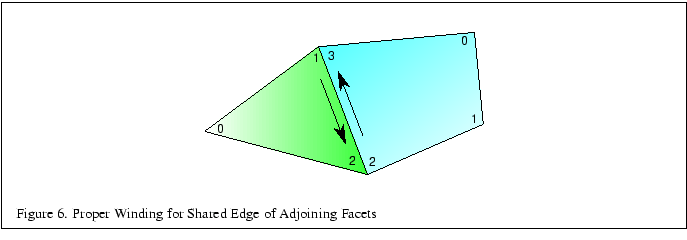Next: 3.3.2 Smooth Shading Up: 3.3 Generating Model Normals Previous: 3.3 Generating Model Normals   Contents

### 3.3.1 Consistent Vertex Winding

Some 3D models come with polygons that are not all wound in a clockwise or counterclockwise direction, but are a mixture of both. Those polygons that are wound inconsistently should have the vertex order reversed. A good way to accomplish this is to find all common edges and verify that neighboring polygon edges are drawn in the opposite order (see Figure 6).To begin rewinding polygons, one polygon must be chosen as correct.'' All neighboring polygons must then be found and made consistent with the correct'' polygon. This repeats recursively for each new correct'' polygon until no more neighboring polygons can be found. If the model is a single closed object, all polygons will now be consistent. However, if the model has multiple unconnected pieces, another polygon that has not yet been tested must be found and the process must be repeated until all polygons have been tested and made consistent.

The above method still leaves a 50-50 chance that the entire object is now wound backwards (assuming an object with half of the facets wound clockwise and half wound counterclockwise). Short of getting a human involved to look at the model, there are ways to check that the normals are pointing outwards. One way is to find the geometric center of the object by computing the object bounding box by finding the maximum and minimum X, Y and Z values, then computing the mid-point of the bounding box. Next, select a vertex that is the maximum distance from this center point and compute the (normalized) vector from the center point to this vertex. Then take the normal of one of the facets that shares the distant vertex and compute the dot product of the two vectors. A positive result indicates that the normals are all correct while a negative result indicates that the normals are all backwards. If the normals are backwards, negate them all and reverse the windings of all facets.

There are still a few pathological cases that may not come out right, such as a model of a room where it is desirable to view the inside walls, but the above method works for most cases.Next: 3.3.2 Smooth Shading Up: 3.3 Generating Model Normals Previous: 3.3 Generating Model Normals   Contents
2001-01-10